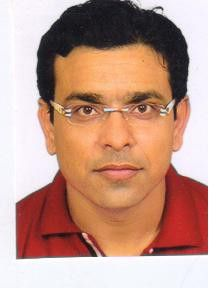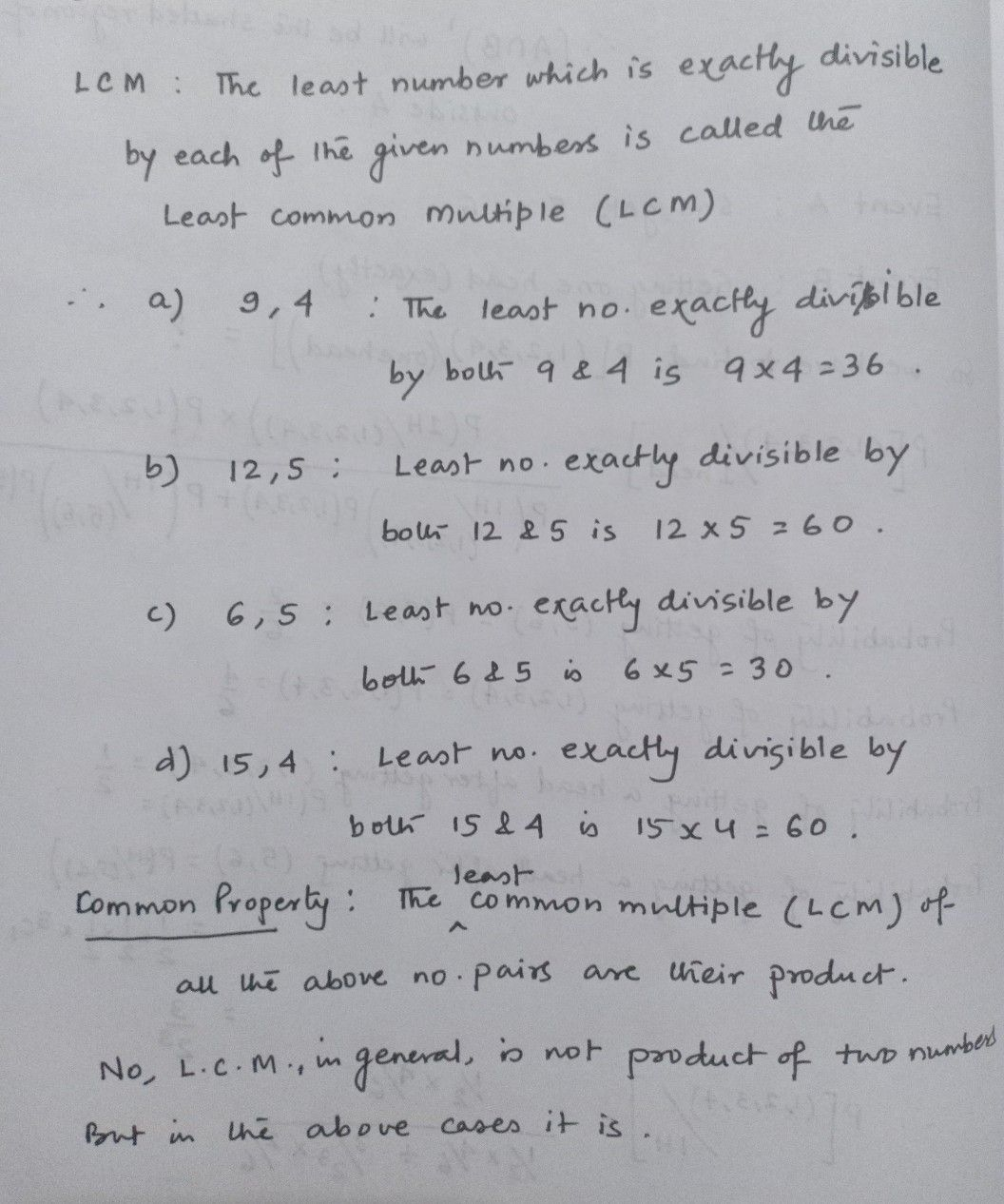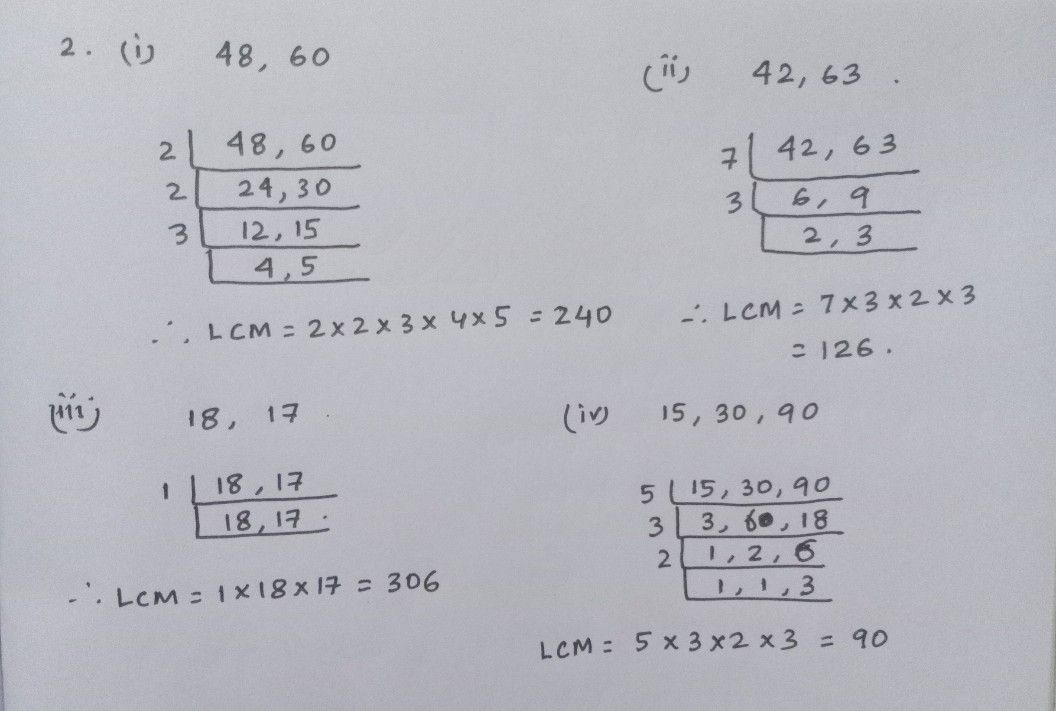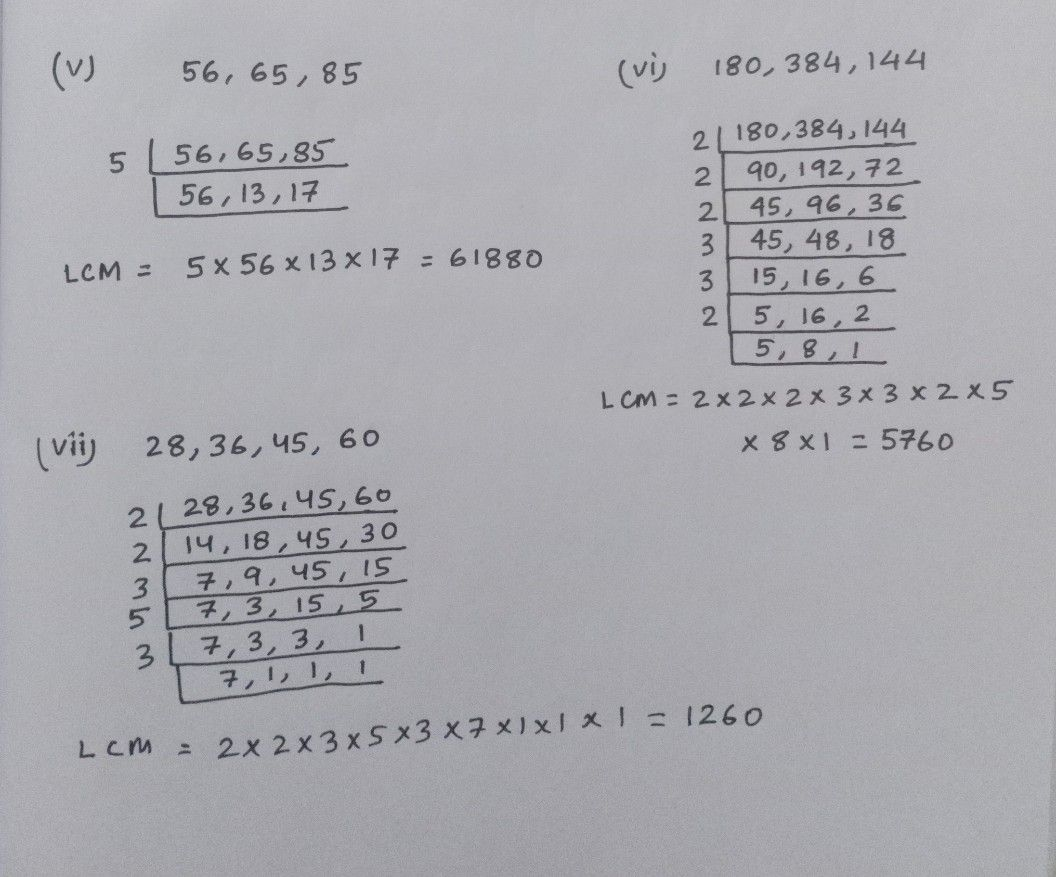Symbol
ProblemEXERCISE Ta) h0 e L 1. Find the $\,_{-.}C_{.} M$ of the following numbers : The i) 9 $11nd4$ ii) 12 and 5 iii) 6 and 5 iv) $15and4$ a) 1: Ooserve a common property in the obtained $CM.s$ $lsL.C$ L.C.M. the product of $1N0$ numbers $C1se3$ 2. Determine the L.C.M $C.M$ of the numbers given $belo1N$ IdaTf ai) a ) v h i) $48.60$ ii) $42.63$ iii) $18,17$ iv) $15,30,90$ v) 56, 65, 85 vi) $180,384$ 144 vii) $28,36,45,60$ 3. i) Find the least number which is exactly divisible by 16, $24q$ and 36. TH ii) Find the least number which is exactly divisible by $14,21,24,30a$ and 42. a 4. What is the smallest number which when divided by $24,36$ and 54 gives a remainder of 5 in each
1st-6th grade
Calculus
SolutionQanda teacher - sanataphyplease post other questions in different post.
RegardsStudent
thank u sir
sir